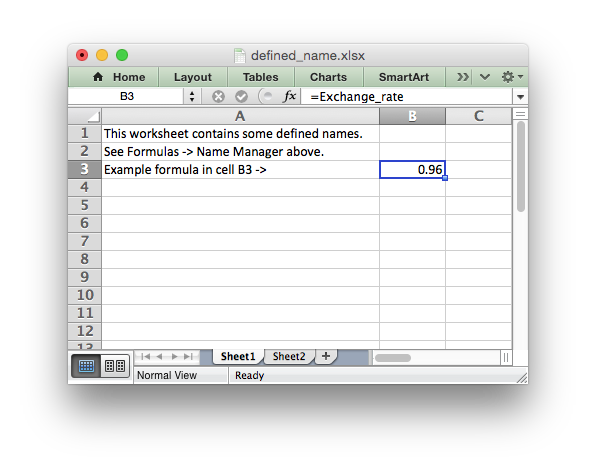# Example: Defined names/Named ranges#

Example of how to create defined names (named ranges) with XlsxWriter.

Defined names are used to define descriptive names to represent a value, a single cell or a range of cells in a workbook or worksheet. See `define_name()`.```##############################################################################
#
# Example of how to create defined names with the XlsxWriter Python module.
#
# This method is used to define a user friendly name to represent a value,
# a single cell or a range of cells in a workbook.
#
# Copyright 2013-2023, John McNamara, jmcnamara@cpan.org
#
import xlsxwriter

workbook = xlsxwriter.Workbook("defined_name.xlsx")

# Define some global/workbook names.
workbook.define_name("Exchange_rate", "=0.96")
workbook.define_name("Sales", "=Sheet1!\$G\$1:\$H\$10")

# Define a local/worksheet name. Over-rides the "Sales" name above.
workbook.define_name("Sheet2!Sales", "=Sheet2!\$G\$1:\$G\$10")

# Write some text in the file and one of the defined names in a formula.
for worksheet in workbook.worksheets():
worksheet.set_column("A:A", 45)
worksheet.write("A1", "This worksheet contains some defined names.")
worksheet.write("A2", "See Formulas -> Name Manager above.")
worksheet.write("A3", "Example formula in cell B3 ->")

worksheet.write("B3", "=Exchange_rate")

workbook.close()
```